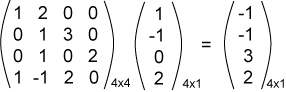# Steps in Multiplication

By the definitions on the previous page, multiplication always involves dot products of rows on the left with columns on the right.

To do any of these calculations:

1. Examine the dimensions of the operands.
• The inner dimensions must be equal: 1xN and NxC.
• "Cancel" the inner dimensions to find the dimensions of the result: 1xC.
• Write an empty result matrix with the correct dimensions.
2. Now fill in each element of the result.
• Each column on the right is flipped onto a row on the left.
• Each element of the result is a dot product.

### QUESTION 13:

Is Ax equal to xA ?